# Plot rms model summaries and predictions

### Load the required libraries

#the regression modelling by F. Harrell
library(rms)

#the extension for plotting the rms summary objects
library(ormPlot)
#> Registered S3 method overwritten by 'ormPlot':
#>   method           from
#>   plot.summary.rms rms

#to modify plots
library(ggplot2)

### Looking at the bundled data

OrmPlot has educ_data bundled. educ_data is used in this vignette to show the functionality of the package. To familiarize with the data:

#show first 6 rows
head(educ_data)
educ_3 Rural sex max_SEP_3 n_siblings cran_rzs height_rzs FW_rzs YOBc YOB
2 Urban Girl Unskilled manual 4 0.6328 -0.1497 -0.5076 -9.224 1940
2 Urban Girl Non-manual 2 0.6386 0.9394 -0.2304 -9.224 1940
3 Rural Girl Unskilled manual 6 0.03449 -0.4342 -0.3903 -8.224 1941
2 Rural Girl Skilled manual 3 0.591 0.44 0.02453 -8.224 1941
1 Rural Girl Unskilled manual 1 1.559 -0.2515 1.6 -8.224 1941
2 Rural Girl Unskilled manual 3 0.03757 0.08076 -0.4793 -7.224 1942
#show variable explanation
help(educ_data)

### Setting up rms datadist

The rms package requires that a datadist object is set up properly. According to datadist documentation: q.effect is a set of two quantiles for computing the range of continuous variables to use in estimating regression effects. Defaults are c(.25,.75), which yields inter-quartile-range odds ratios

#q.effect determines for what range the odds ratios are given on plots
dd <- datadist(educ_data, q.effect = c(0.5, 0.75))
#set it also to options
options(datadist="dd")

### Creating a model

#see help(orm) for further info
orm_model<-orm(educ_3 ~ Rural + sex + n_siblings + cran_rzs + height_rzs +
FW_rzs + YOBc + (YOBc * sex) + (YOBc * Rural), data = educ_data)

### Plotting the model predictions with CI

The main advantage of using ormPlot is that you get plots with confidence intervals shown on the plot.

The simplest way is to predict for only one value. Plotting returns a customizable ggplot object.

plot(orm_model, cran_rzs)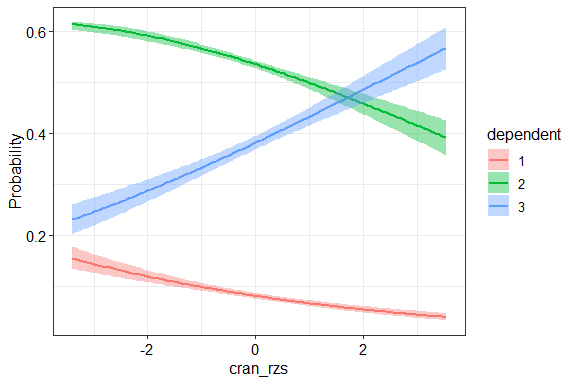For more complex models specify facet column and rows.

plot(orm_model, cran_rzs,  Rural, sex)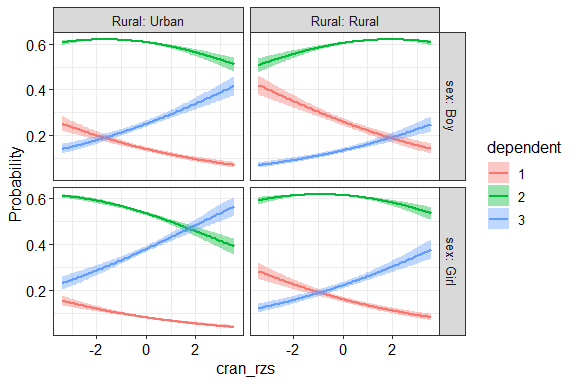You can easily set custom labels.


p<-plot(orm_model, cran_rzs,  Rural, sex,
xlab = "Cranial volume (residuals to age an birth date)",
facet_labels = list(Rural = c("Urban", "Rural"),sex=c("Male","Female")))

colors <- c("#4a9878", "#0a191e", "#d8b65c")
educ_names <- c("Primary", "Secondary", "Tertiary")

# further modifing like any other ggplot
final_plot<-p + labs(color = "Education", fill = "Education") +
scale_color_manual(values = colors, labels = educ_names) +
scale_fill_manual(values = colors, labels = educ_names)

final_plot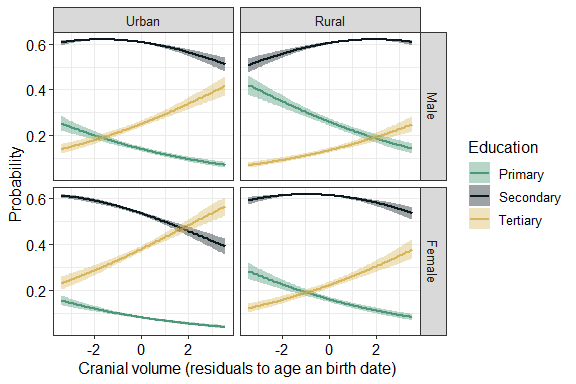Save like any ggplot graph.

ggsave("educ_cran.svg",final_plot, height = 8 ,width = 8)

### Plotting the model summary

The easiest way is to just plot the summary object

forestplot(summary(orm_model))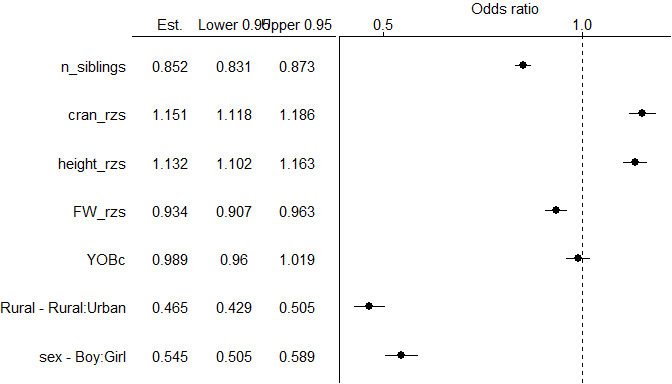If this does not look nice enough you can also get ggplot2 objects to customize to your needs. The best way to get customizable plots is to specify return_ggplots=TRUE

# you can use also use plot instead of forestplot
plots<-forestplot(summary(orm_model), return_ggplots=T)
plots[]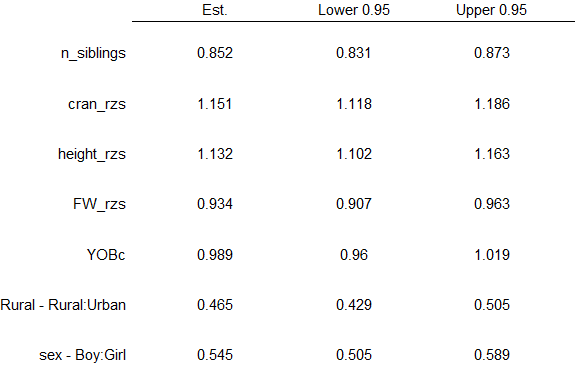plots[]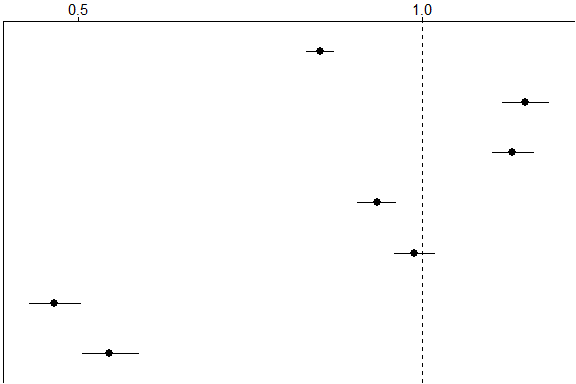These can be joined using the join_ggplots command. You can edit the plots as any ggplot plot


p1 <- plots[] +
scale_x_discrete(labels=c("Mean", "Lower CI", "Upper CI"),
position = "top",
name = NULL)
#> Scale for 'x' is already present. Adding another scale for 'x', which will
#> replace the existing scale.

# the x axis is actually y axis because the cordinates are flipped with coord_flip()
p2 <- plots[] + scale_y_continuous(breaks = c(0.5, 0.7, 0.9, 1.1),
position = "right")
#> Scale for 'y' is already present. Adding another scale for 'y', which will
#> replace the existing scale.

forestplot<-join_ggplots(p1,p2)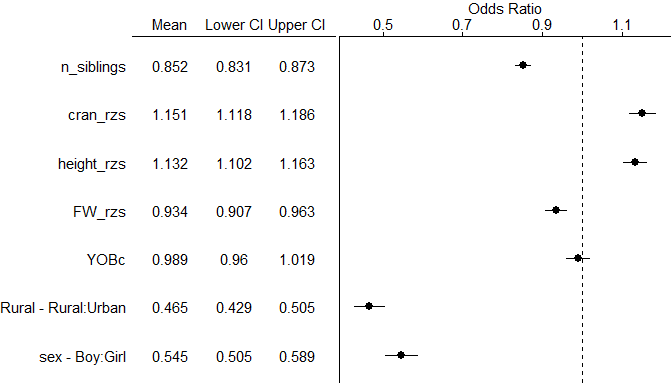To save as svg fur further editing just use ggsave from ggplot2

ggsave("forestplot.svg",forestplot)